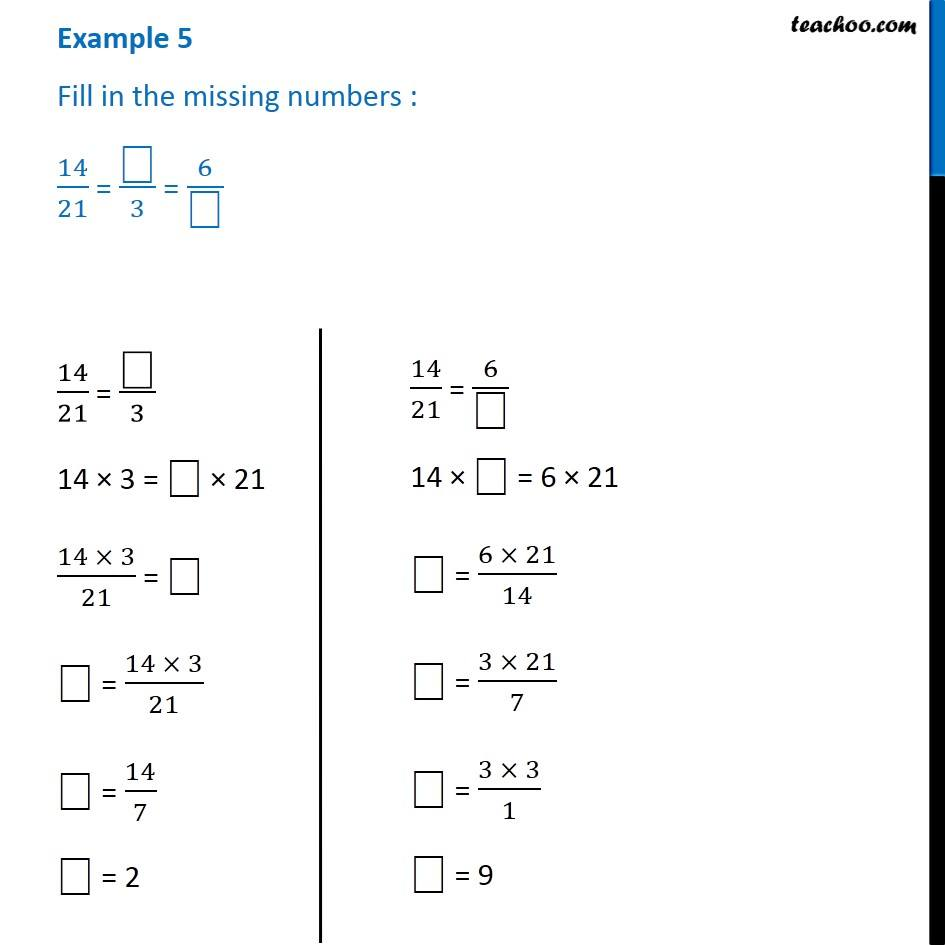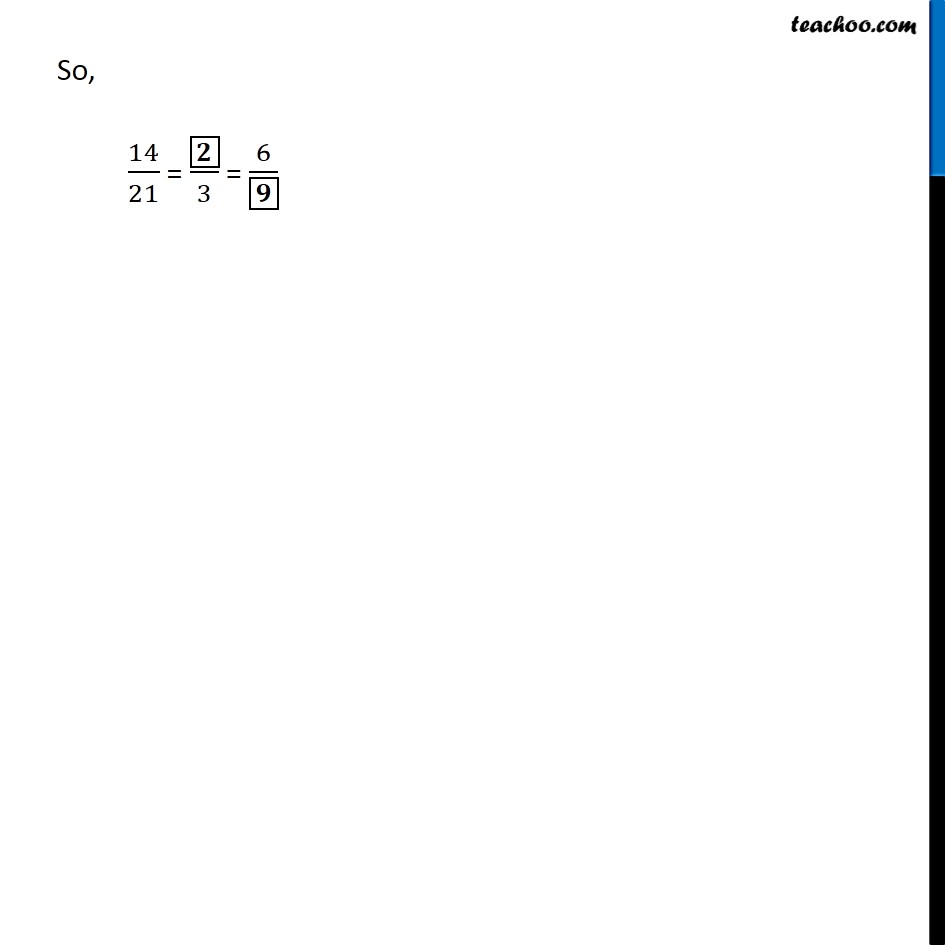Examples

Chapter 12 Class 6 Ratio and Proportion
Serial order wiseLearn in your speed, with individual attention - Teachoo Maths 1-on-1 Class

### Transcript

Example 5 Fill in the missing numbers : 14/21 = "⎕" /3 = 6/"⎕" 14/21 = "⎕" /3 14 × 3 = "⎕" × 21 (14 × 3)/21 = "⎕" "⎕" = (14 × 3)/21 "⎕" = 14/7 "⎕" = 2 14/21 = 6/"⎕" 14 × "⎕" = 6 × 21 "⎕" = (6 × 21)/14 "⎕" = (3 × 21)/7 "⎕" = (3 × 3)/1 "⎕" = 9 So, 14/21 = ▭𝟐/3 = 6/▭𝟗